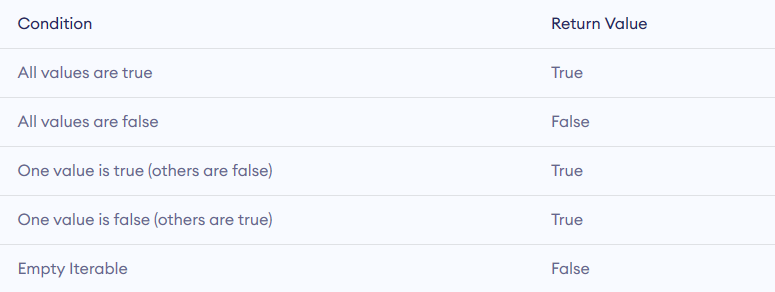## Example program for Any function in python - Python Example Program

Any function in python programming number of iterable is used and return the true if any element of any iterable is true or return the false.Any function in python

## Syntax of any function in python

`any(iterable)`

Any function in python take iterable (string, dictionary etc.) as a Parameters.

Return : -

it's Return the boolean value.

•  True if any one element is true
•  Return the false if all the element are false.

# Example program for any() function in python : -

```l = [1, 3, 4, 0]
print(any(l))

l = [0, False]
print(any(l))

l = [0, False, 5]
print(any(l))

l = []
print(any(l))```

```True
False
True
False```

## Example program for any function on Python Strings

```s = "This is best"
print(any(s))

# 0 is False
# '0' is True since its a string character
s = '000'
print(any(s))

# False since empty iterable
s = ''
print(any(s))```